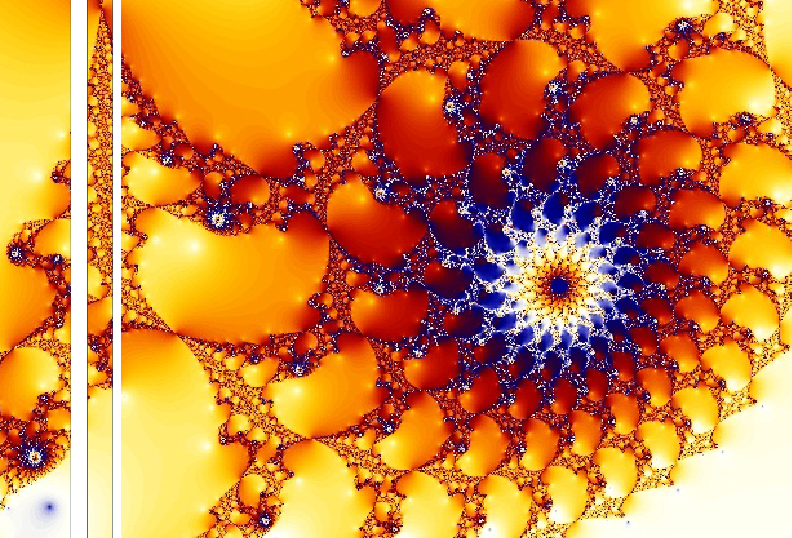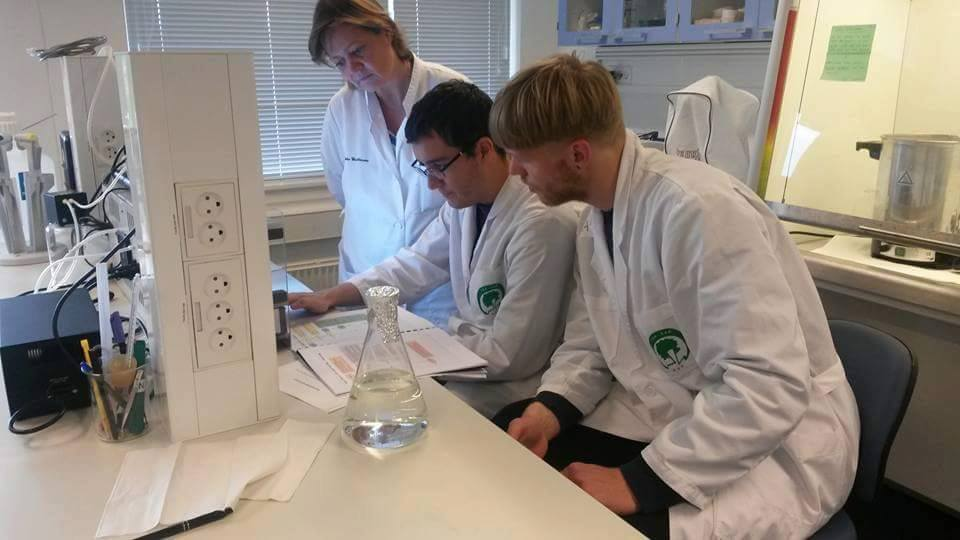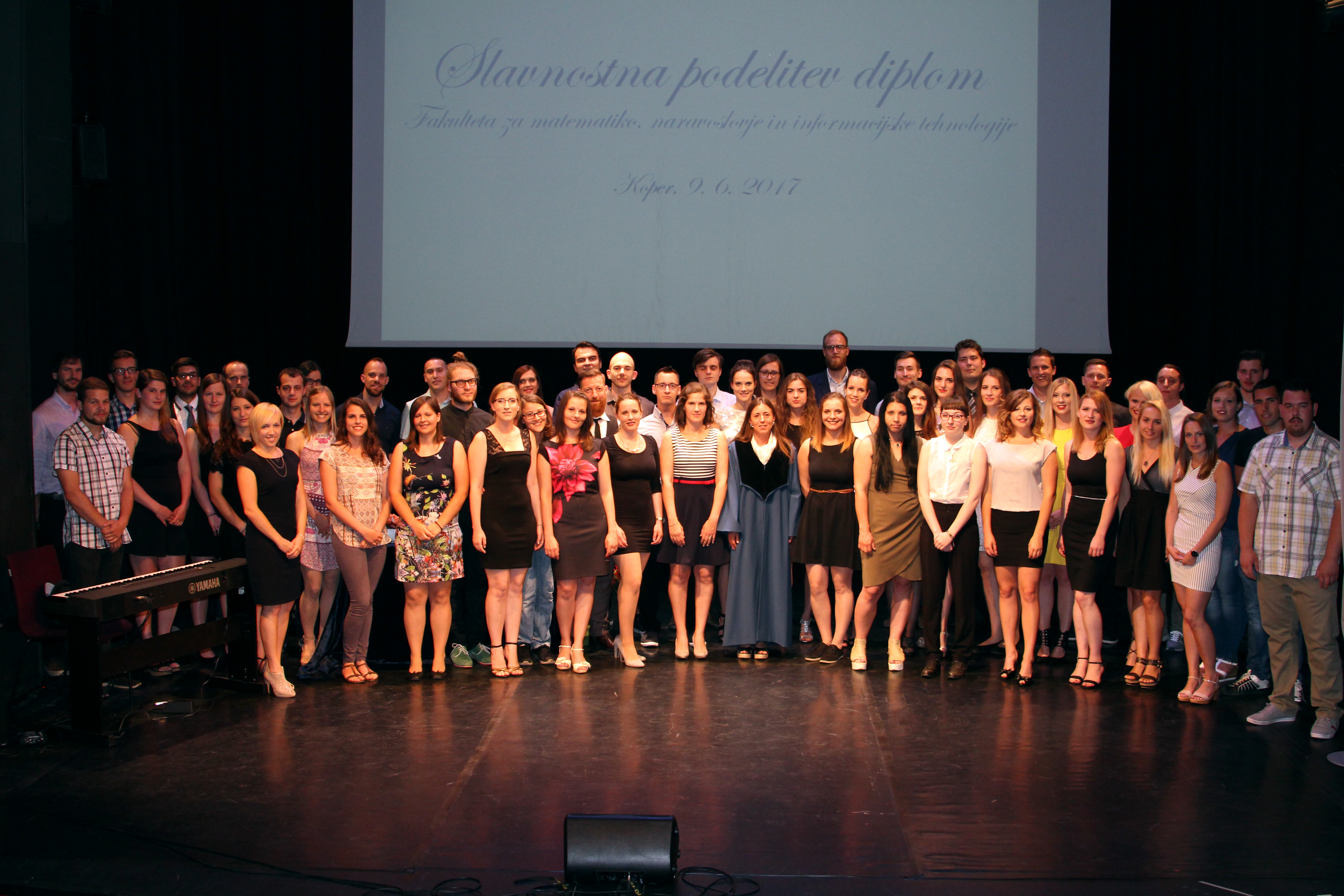Univerza na Primorskem Fakulteta za matematiko, naravoslovje in informacijske tehnologijeSI | ENRaziskovalni matematični seminar - Arhiv

 2022 2021 2020 2019 2018 2017 2016 2015 2014 2013 2012 2011 2010
 1 2 3 4 5 6 7 8 9 10 11 12
Datum in ura / Date and time: 27.7.21
(17:00 -- 18:00)
Predavalnica / Location: Zoom
Predavatelj / Lecturer: Sarobidy Razafimahatratra (University of Regina, Canada)
Naslov / Title: The Erdos-Ko-Rado theorem for transitive groups
Vsebina / Abstract:

A set of permutations $\mathcal{F}$ of a finite transitive group $G\leq \sym(\Omega)$ is \emph{intersecting} if any two permutations in $\mathcal{F}$ agree on an element of $\Omega$. The transitive group $G$ is said to have the \emph{Erd\H{o}s-Ko-Rado (EKR) property} if any intersecting set of $G$ has size at most $\frac{|G|}{|\Omega|}$.

The alternating group $Alt(4)$ acting on the six $2$-subsets of $\{1,2,3,4\}$ is an example of groups without the EKR property. Hence, transitive groups need not have the EKR property.    Given a transitive group $G\leq \sym(\Omega)$, we are interested in finding the size and structure of the largest intersecting sets in $G$. In this talk, we will give an overview of the EKR-theory for transitive groups and present some recent development in this area.

Join Zoom meeting here:

https://upr-si.zoom.us/j/86104965231?pwd=SFZoMWFpbTN4SmtCMXc1OVVQQmVEQT09

We are looking forward to meeting at the video conference.

See you there!

Datum in ura / Date and time: 26.7.21
(11:00 -- 12:00)
Predavalnica / Location: Zoom
Predavatelj / Lecturer: Milad Ahanjideh (Bogazici University, Turkey )
Naslov / Title: On intersecting sets of groups
Vsebina / Abstract:

Let $\Omega$ be a finite set and $G$ be a permutation group on it.

A subset $A$ of $G$ is \textit{intersecting} if for every $\delta,~\tau \in A$, they agree at some points, i.e. there exists $x \in \Omega$ such that $\delta(x)=\tau(x)$.

In this talk, I will give an analog of the classical Erd\H{o}s–Ko–Rado theorem for intersecting sets of a group.  I will present a history and overview of some of the results that have been proved on this subject. Finally,  I will discuss some of my results about intersecting sets of the general linear group and its subgroups.

Join Zoom meeting here:

https://upr-si.zoom.us/j/83882798074?pwd=VTdlLzVmRExBWGdwY1pTZFAvUkZMdz09